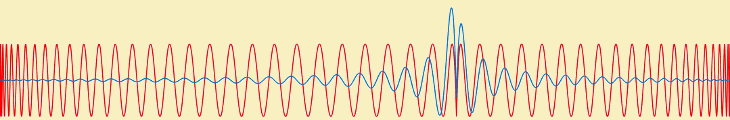## Approximation theory

### Description

This project is about how to approximate a continuous function using a discrete basis of simpler functions. The practical applications of this idea are far-reaching: having a discrete representation makes functions easier to store (think mp3 or jpeg compression), easier to evaluate, and easier to compute with (think differentiation, rootfinding, etc.).

You have already seen this basic idea in Numerical Analysis II, where we studied polynomial interpolation and least-squares approximations. But we only scratched the surface of the subject known as "Approximation Theory". This project will give you an opportunity to explore more deeply into this important and fun area of Numerical Analysis.

There are many different directions that this project could take. For example, piecewise-polynomial interpolants (such as cubic splines), minimax approximations, Bezier curves, or trigonometric polynomials (leading to the fast Fourier transform and image compression). We will start by reading and discussing some of these types of approximation, then each student will be encouraged to specialise on one area - going into more detail and (ideally) implementing the methods numerically in Python.The error curves $f(x)-p(x)$ for two different polynomial approximations of degree 100 to the function $f(x)=|x-\frac{1}{4}|$. The first is the "minimax" polynomial approximation (red) and the second is the interpolant at the Chebyshev nodes (blue).

### Prerequisites & corequisites

Numerical Analysis II is an essential prerequisite. Reasonable working competency in Python would definitely be an advantage.

### Resources

You may want to read the essay Six Myths of Polynomial Interpolation and Quadrature (by L.N. Trefethen). The History of Approximation Theory has a list of people and links to seminal papers. Some other articles of interest include: B-splines and geometric design (SIAM News), From Bézier to Bernstein (AMS feature column), The Chebyshev Equioscillation Theorem (R. Mayans), Explained: The Discrete Fourier Transform (MIT News), Image Compression: How Math Led to the JPEG2000 Standard (SIAM).

For the mathematical details, many textbooks on Numerical Analysis cover some aspects of Approximation Theory. For example, An Introduction to Numerical Analysis (Suli and Mayers); Numerical Analysis (Burden and Faires, many editions!); Numerical Analysis (L.R. Scott); An Introduction to Numerical Analysis (K. Atkinson).

email: A Yeates